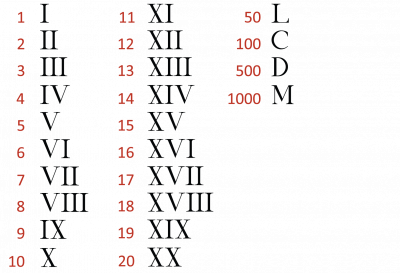The ancient Romans had a special way of writing numbers. They used capital letters! Roman numbers are called numerals. The Romans put different letters together to make different numbers.If you put a letter after a larger one it means you add it

Here is an example:

LV = 55

This means 50 (L) + 5 (V) = 55

50 + 5 = 55

If you put a letter before a larger one it means you take it away

Here is an example:

XC = 90

This means you take 10 (X) away from 100 (C)

100 − 10 = 90

Let's practise some easy sums:

Example 1: LV + C = ?

Answer: 55 + 100 = 155

Example 2: CM − D = ?

Answer: 900 − 500 = 400